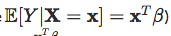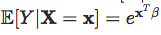GLM：链接与分发

X  =  Ç（1，2，3，4，5）
Ý  =  Ç（1，2，4，2，6）
base  =  data.frame（x，y）regNId  =  glm（y ~ x，family = gaussian（link = “ identity”），data = base）
regNlog  =  glm（y ~ x，family = gaussian（link = “ log”），data = base）
regPId  =  glm（y ~ x，family = poisson（link = “ identity”），data = base）
regPlog  =  glm（y ~ x，family = poisson（link = “ log”），data = base）
regGId  =  glm（y ~ x，family = Gamma（link = “ identity”），data = base）
regGlog  =  glm（y ~ x，family = Gamma（link = “ log”），data = base）
regIGId  =  glm（y ~ x，family = inverse.gaussian（link = “ identity”），data = base）
regIGlog  =  glm（y ~ x，family = inverse.gaussian（link = “ log”），data = base

regTwId  =  glm（y ~ x，family = tweedie（var.power = 1.5，link.power = 1），data = base）
regTwlog  =  glm（y ~ x，family = tweedie（var.power = 1.5，link.power = 0），data = base）

darkcols  =  brewer.pal（8，“ Dark2”）

abline（regNId，col = darkcols [ 1 ]）
abline（regPId，col = darkcols [ 2 ]）
abline（regGId，col = darkcols [ 3 ]）
abline（regIGId，col = darkcols [ 4 ]）
abline（regTwId，lty = 2）û = SEQ（0.8，5.2，通过= 0.01）

line（u，predict（regGlog，newdata = data.frame（x = u），type = “ response”），col = darkcols [ 3 ]）Vgamma  =  SEQ（- 0.5，3.5，通过= 0.05）
Vpente  =  Vectorize（pente）（Vgamma）erreur = function（gamma）predict（glm（y ~ x，family = tweedie（var.power = gamma，link.power = 1），data = base），newdata = data.frame（x = 1），type = “ 回应“）- y [ x == 1 ]
Verreur  =  Vectorize（erreur）（Vgamma）
plot（Vgamma，Verreur，type = “ l”，lwd = 3，ylim = c（- .1，.04），xlab = “ power”，ylab = “ error”）
abline（h = 0，lty = 2）Vpente  =  Vectorize（pente）（Vgamma）
plot（Vgamma，Vpente [ 1，]，type = “ l”，lwd = 3）plot（Vgamma，Vpente [ 1，]，ylim = c（0，.8），type = “ l”，lwd = 3，xlab = “ power”，ylab = “ slope”）erreur = function（gamma）predict（glm（y ~ x，family = tweedie（var.power = gamma，link.power = 0），data = base），newdata = data.frame（x = 1），type = “ 回应“）- y [ x == 1 ]
Verreur  =  Vectorize（erreur）（Vgamma）
plot（Vgamma，Verreur，type = “ l”，lwd = 3，ylim = c（.001，.32），xlab = “ power”，ylab = “ error”）• 博文量
188
• 访问量
112637